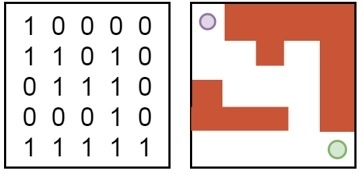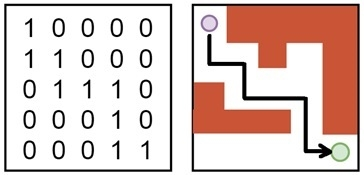Rat in a Maze Problem

Data StructureBacktracking AlgorithmsAlgorithms

In this problem, there is a given maze of size N x N. The source and the destination location is top-left cell and bottom right cell respectively. Some cells are valid to move and some cells are blocked. If one rat starts moving from start vertex to destination vertex, we have to find that is there any way to complete the path, if it is possible then mark the correct path for the rat.

The maze is given using a binary matrix, where it is marked with 1, it is a valid path, otherwise 0 for a blocked cell.

NOTE: The rat can only move in two directions, either to the right or to the down.

Input and Output

Input:
This algorithm will take the maze as a matrix.
In the matrix, the value 1 indicates the free space and 0 indicates the wall or blocked area.In this diagram, the top-left circle indicates the starting point and the bottom-right circle indicates the ending point.
Output:
It will display a matrix. From that matrix, we can find the path of the rat to reach the destination point.Algorithm

isValid(x, y)

Input: x and y point in the maze.

Output: True if the (x,y) place is valid, otherwise false.

Begin
if x and y are in range and (x,y) place is not blocked, then
return true
return false
End

solveRatMaze(x, y)

Input− The starting point x and y.

Output − The path to follow by the rat to reach the destination, otherwise false.

Begin
if (x,y) is the bottom right corner, then
mark the place as 1
return true
if isValidPlace(x, y) = true, then
mark (x, y) place as 1
if solveRatMaze(x+1, y) = true, then //for forward movement
return true
if solveRatMaze(x, y+1) = true, then //for down movement
return true
mark (x,y) as 0 when backtracks
return false
return false
End

Example

#include<iostream>
#define N 5
using namespace std;

int maze[N][N]  =  {
{1, 0, 0, 0, 0},
{1, 1, 0, 1, 0},
{0, 1, 1, 1, 0},
{0, 0, 0, 1, 0},
{1, 1, 1, 1, 1}
};

int sol[N][N];         //final solution of the maze path is stored here
void showPath() {
for (int i = 0; i < N; i++) {
for (int j = 0; j < N; j++)
cout << sol[i][j] << " ";
cout << endl;
}
}

bool isValidPlace(int x, int y) {      //function to check place is inside the maze and have value 1
if(x >= 0 && x < N && y >= 0 && y < N && maze[x][y] == 1)
return true;
return false;
}

bool solveRatMaze(int x, int y) {
if(x == N-1 && y == N-1) {       //when (x,y) is the bottom right room
sol[x][y] = 1;
return true;
}

if(isValidPlace(x, y) == true) {     //check whether (x,y) is valid or not
sol[x][y] = 1; //set 1, when it is valid place
if (solveRatMaze(x+1, y) == true)       //find path by moving right direction
return true;
if (solveRatMaze(x, y+1) == true)         //when x direction is blocked, go for bottom direction
return true;
sol[x][y] = 0;         //if both are closed, there is no path
return false;
}
return false;
}

bool findSolution() {
if(solveRatMaze(0, 0) == false) {
cout << "There is no path";
return false;
}
showPath();
return true;
}

int main() {
findSolution();
}

1 0 0 0 0
1 1 0 0 0
0 1 1 1 0
0 0 0 1 0
0 0 0 1 1